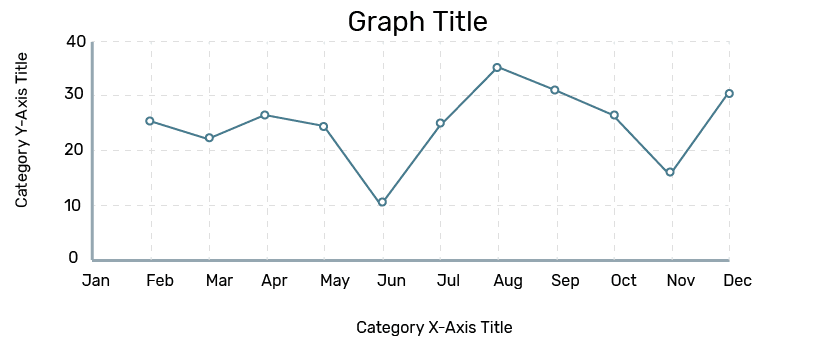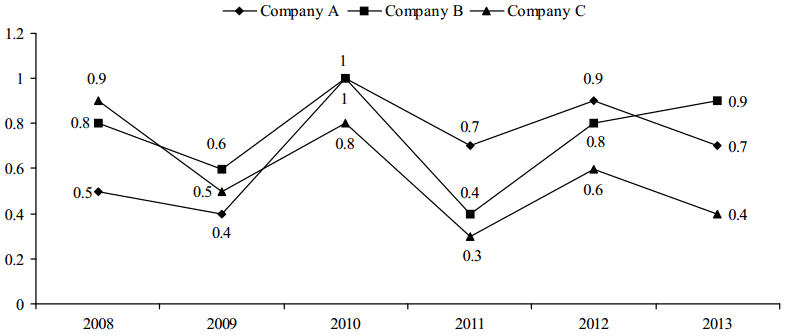UPSC  >  Line Graphs: Introduction & Examples (with Solutions)

# Line Graphs: Introduction & Examples (with Solutions) - CSAT Preparation - UPSC

## What is a Line Graph?

Line Graph is the innovative version of Bar Graph representation.

If we connect the upper point of the first Bar to the upper point of the second Bar and then tie these dots, we will get a line. Repeating the procedure gives us the Line Graph representation. Line graphs and bar graphs are easy to comprehend. A Line Graph looks like this:Line Graph

### Sample Question

The following line graph shows the ratio of expenditure to income of three companies A, B and C during the period 2008–2013.Reading the headings is important, otherwise, you will not be able to understand what these lines are all about.

Along Y-Axis are the ratios. Along X-Axis are the years. In between are the lines.

### Learn a few things from the heading:

1. For Company A in 2008, if Expenditure is Rs 0.9, then Income will be Rs 1, and so on.
2. It’s Expenditure to Income Ratio expressed as E:I and not Income to Expenditure.
3. To have a Profit, Expenditure is to be less than Income. The reverse is for Loss.
4. Profit and Loss percentages are calculated using the formulas for the same.
• Profit = Income – Expenditure
• Profit Percentage = [Profit/Expenditure]*100
• Loss = Expenditure – Income
• Loss Percentage = [Loss/Expenditure]*100
5. The lower is the E:I ratio, higher is the profit.

The questions of Expenditure and Income seem difficult to solve. But, let’s apply the above mentioned points to solve the questions in no time!

## Examples

Q1. In which of the following years is the percentage loss/profit of Company C the maximum?

 2008
 2009
 2010
 2011
 2012

Solution:

From point no. 5 above, we conclude that profit is maximum when E:I is minimum which is 0.3 in 2011.

Q2. If the expenditure of Company A in 2008 and 2009 together is Rs 60 lakhs, then what is its income in 2008 and 2009 together?

 Rs 120 lakhs
 Rs 150 lakhs
 Rs 66.66 lakhs
 None of these

Solution:

E:I for Company A in 2008 and 2009 is 0.5 and 0.4. This means for Rs 0.5 Expenditure in 2008, Income is Rs 1 in 2008 and for Rs 0.4 Expenditure in 2009, Income is Rs 1 in 2009. But combined Expenditure of 60 lakhs is given. So, ratios being different, it’s not possible to calculate the Income from the combined expenditure. The answer is .

Q3. If the expenditure of Company B in 2008 and 2012 together is Rs 60 lakhs then what is its income in 2008 and 2012 together?

 Rs 66.66 lakhs
 Rs 75 lakhs
 Rs 48 lakhs
 96 Rs lakhs

Solution:

E:I for 2008 and 2012 is 0.8 and 0.8. Ratios being the same, combined Income from the combined Expenditure can be calculated. Income = E/0.8 = 60/0.8 = 75 lakhs.

Q4. In which of the years does Company C gain 100% profit?

 2008
 2009
 2010
 2011
 None of these

Solution:

For 100% profit, E:I ratio must be 0.5 so that I = E/0.5 = 2E. It’s in 2009.

Q5. What is the percentage decrease in the percentage profit of Company C from 2009 to 2010?

 75%
 300%
 62.5%
 160%
 None of these

Solution:

E:I of Company C in 2009 = 0.5:1
Profit = 1-0.5 = 0.5
Percentage profit of profit of Company C in 2009 =[0.5/0.5]*100 = 100%
E:I of Company C in 2010 = 0.8:1
Profit = 1-0.8 = 0.2
Percentage profit of profit of Company C in 2009 =[0.2/0.8]*100 = 25%
Percentage decrease = 75%.

The document Line Graphs: Introduction & Examples (with Solutions) | CSAT Preparation - UPSC is a part of the UPSC Course CSAT Preparation.
All you need of UPSC at this link: UPSC

## CSAT Preparation

197 videos|151 docs|200 tests

## FAQs on Line Graphs: Introduction & Examples (with Solutions) - CSAT Preparation - UPSC

 1. What is a line graph?A line graph is a type of chart that displays data as a series of points connected by line segments. It is used to track changes over time and show the relationship between two or more variables.
 2. How is a line graph constructed?To construct a line graph, you need to have data points for different time periods or categories. Plot the data points on the graph, with time or categories on the x-axis and the corresponding values on the y-axis. Then, connect the points with straight lines to show the trend or pattern.
 3. What are the advantages of using a line graph?There are several advantages of using a line graph. Firstly, it allows for easy visualization and interpretation of trends and patterns. Secondly, it can show the relationship between two or more variables. Additionally, it is effective in displaying data changes over time. Lastly, it is suitable for representing continuous data.
 4. How can I interpret a line graph?To interpret a line graph, you need to examine the direction and slope of the lines. If the line is going upwards, it indicates an increase in the variable being measured. Conversely, if the line is going downwards, it indicates a decrease. The steepness of the line also represents the rate of change.
 5. Can a line graph be used to compare multiple sets of data?Yes, a line graph can be used to compare multiple sets of data. Each set of data can be represented by a different line on the graph. By comparing the lines, you can observe the differences and similarities between the variables being measured.

## CSAT Preparation

197 videos|151 docs|200 testsExplore Courses for UPSC exam### How to Prepare for UPSC

Read our guide to prepare for UPSC which is created by Toppers & the best Teachers
Signup to see your scores go up within 7 days! Learn & Practice with 1000+ FREE Notes, Videos & Tests.
10M+ students study on EduRev
Track your progress, build streaks, highlight & save important lessons and more!
Related Searches

,

,

,

,

,

,

,

,

,

,

,

,

,

,

,

,

,

,

,

,

,

;